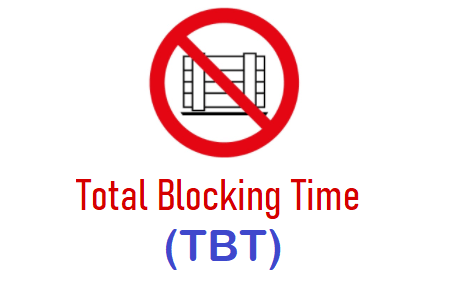# Total Blocking TimeTotal blocking time defines as the sum of all the long tasks (=Long Task processing Time – 50ms) between First Contentful Paint and Time To Interactive period which blocks the main thread and prevents the user from interacting with the web page.

In the UI Performance Testing term, the main thread task that takes more than 50 milliseconds is termed as ‘Long Task’.

## How to calculate Block Time?

Let’s consider the main thread task (say A) taking 250ms to complete. As per the above definition, this task will be considered as ‘Long Task’ because it is taking more than 50ms. To calculate the block time, subtract 50ms from the processing time of the task and that will provide the block time of that particular task.

Block Time (in ms) = Task Processing Time (in ms) – 50

=> Block Time = 250 – 50;
=> Block Time = 200 ms

So, 200ms is the block time of task A. Since there could be multiple long tasks performed by the main thread to load a web page so calculate the block time of each long task using the above formula and then sum up all the block time to get the ‘Total Blocking Time’. Refer to the below figure:

Processing Time of

1. Task A => 250 – 50 = 200ms
2. Task B => 130 – 50 = 80ms
3. Task C => 60 – 50 = 10ms
4. Task D => 110 – 50 = 60ms

Total Blocking Time = 200 + 80 + 10 + 60
Total Blocking Time = 350ms

## What is the ideal value of Total Blocking Time?

TBT standard as per Google Lighthouse is:

## How to improve TBT?

1. Optimize JavaScript